# Magnetic field on the axis of a circular current loop | cbse24

Consider a circular loop of wire of radius a and carrying current I, as shown in figLet the plane of the loop be perpendicular to the plane of the paper. We going to find field B at an axial point P at a distance of r from the centre C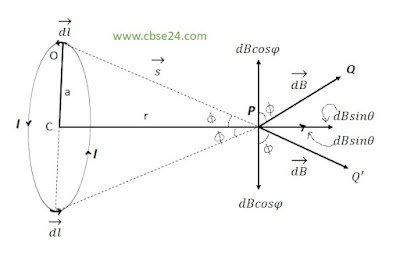The magnetic field on the axis of a circular current loop

Consider a current element dl at the top of the loop. It has an outward coming current.

If s be the position vector of point P relative to the element dl, the Biot-savert law , the field at point P due to the current element is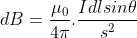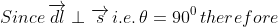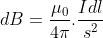dB resolved into two rectangular components.
1. dBsinΦ along the axis
2. dBcosΦ perpendicular to the axis
∴ The total magnetic field at the point P in the direction CP is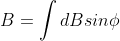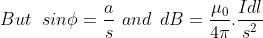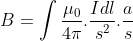Since μo and I are constant, and s and a is the same for all points on the circular loop, we have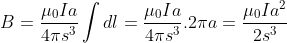{∵ ∫ dl = circumference=2πa}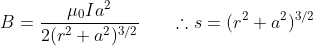If the coil consists of N turns, then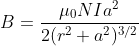*